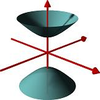#### You may also like### A Close Match

Can you massage the parameters of these curves to make them match as closely as possible?### Prime Counter

A short challenge concerning prime numbers.### The Right Volume

Can you rotate a curve to make a volume of 1?

##### Age 16 to 18 ShortChallenge Level

Our thanks go to azerbajdzan for correcting an error in the original solution to this challenge

The first part of this journey consists of a sequence of forward motions followed by 90 degree turns. Although we could just halve repeatedly to find the number of turns, we might consider the possibility that the numbers in the problems might change, so look for a general proceduce for finding the number of motions.

In doing this, we need to be careful about the number of forward turns and note that the WHILE(DISTANCE > 0.001) condition is evaluated after each halving.

The forward motion, if it occurs, following the $n$th turn will be of distance $1000\times 2^{-n}$.This will occur for each $n$ for which

$1000\times 2^{-n}> 0.001$
Taking logs gives

$\log(1000) -n\log(2)> \log(0.001)$
Rearranging gives

$n < \frac{\log(1000)-\log(0.001)}{\log(2)}=\frac{\log(1000000)}{\log{(2)}}\approx 19.93$

This first becomes invalid AFTER after 20 turns. Thus, the path of the robot is determined by $20$ motions. The directions that the robot travels in these 20 motions are alternately NE, SE, SW, NW, .... Since there are 20 motions in total there are 5 motions in each of these directions.

Let $T$ be the total distance travelled NE. Then

$T= 1000\left(\frac{1}{2^0}+\frac{1}{2^4}+\frac{1}{2^8}+\frac{1}{2^{12}}+\frac{1}{2^{16}}\right)$

Note that the distance SE will be half of this, since each motion is half the length of the preceding NE motion. Similarly, total distance SW will be one quarter of $T$; total distance NW will be one eight of $T$. Each of these motions contributes a component in the $x$-direction and component in the $y$-direction.

The final coordinates will therefore be
$$(x, y) = \left(\frac{T}{\sqrt{2}}\left(1+\frac{1}{2}-\frac{1}{4}-\frac{1}{8}\right),\frac{T}{\sqrt{2}}\left(1-\frac{1}{2}-\frac{1}{4}+\frac{1}{8}\right)\right)=\left(\frac{9T}{8\sqrt{2}}, \frac{3 T}{8\sqrt{2}}\right)$$